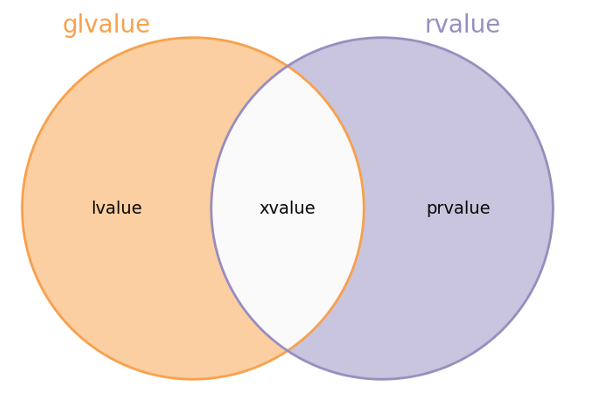## 1 背景(Background)

### 1.1 在其它语言中的历史

An L-value represents an area of the store of the computer. We call this a location…
Some locations are addressable but some are not.
The two essential features of a location are that it has a content—i.e. an associated R-value

### 1.2 在C++中的发展

C++规范对这两个术语的精确措辞很难，但是有助于解决一些已知的规范问题（与右值引用有关）。Stroustrup原先不打算做出更改，但是，CWG大多数人都不同意并坚持认为，必须有一些更改或使用新术语来解决已知问题和使规范保持一致。

#### 每个值都有两个独立的属性：

• 具有身份（has identity) –比如一个指针，一个地址，用户能决定这两份拷贝是具有身份的。
• 可移动(can safely be moved from)–就是允许移动之后，脱离拷贝的来源，其状态有效。

#### 基于这两个属性，就有了三种不同的组合结果：

• 具有身份+不可移动
• 具有身份+可移动
• 没有身份+可移动• 具有身份+不可移动 –> lvalue
• 具有身份+可移动 –> xvalue
• 没有身份+可移动 –> prvalue

## 2 基本概念(Basic concepts)

Every expression is either an lvalue or an rvalue. 每个表达式不是左值，就是右值。

lvalue 一个左值(在历史上，因为左值可能出现在赋值表达式的左侧)指定一个函数或者对象。【例子：如果p是一个表达式的指针类型，那么*p就是一个左值表达式，它引用p所指的一个对象或者函数。另一个例子：调用一个函数，它的返回值类型是左值引用，那么函数的结果是左值。】
xvalue xvalue (an “eXpiring”过期的值)。它也引用一个对象，该对象通常接近它的生命周期(以至于它的资源可被移动)。xvalue是某些包含右值引用的表达式【示例：函数返回类型为右值引用的是xvalue。】
glvalue (“generalized” lvalue， 广义的左值)，它是一个lvalue或者xvalue
rvalue （在历史上，因为右值可能出现在赋值表达式的右侧）是一个xvalue，一个临时对象或子对象，或没有与任何对象关联的值。
prvalue (“pure” rvalue) 是一个ralue，但不是xvalue. 【比如函数的调用结果，其返回值不是一个引用，那就是prvalue；再比如字面的12,7.e5, 或者ture 都是prvalue】lvalue 具有一个地址，并且程序可访问该地址。比如变量，指针，类成员函数 …
xvalue 具有一个地址，但程序不再能访问。
glvalue　 具有地址
prvalue 它没有一个地址
rvalue 它没有一个地址，或者它有地址但是程序不再能访问

## 3 值分类示例(Examples)

### 3.1 解释lvalue和prvalue

``````int y;
y = 10;   // 一个常见的表达式
``````

• 作为右操作数的数字10，，是ravlue,　更是prvalue.
• 作为左操作数的变量y，是glvalue,　更是lvalue.

1. 右边的数字10是rvalue，因为它是没有内存地址的。更精确的说，它是prvalue(由于它是rvalue且不是xvalue)。
2. 左边的变量 `y`是glvalue， 因为它是一个变量，它有内存地址。更精确的说，它是lvalue(由于这个变量不是临时变量,　程序可继续访问它的地址，意味着它不是xvalue)

#### 3.1.1 赋值的左操作数需要lvalue

``````10 = y;    // error!
``````

``````error: lvalue required as left operand of assignment

``````

``````int i = 2;
i * 4 = 7;  // error!
``````

``````int setValue()
{
return 6;
}
// ... somewhere in main() ...
setValue() = 3; // error!
``````

``````int my = 100;

int& set_my_value()
{
return my;
}
// ... somewhere in main() ...
set_my_value() = 400; // OK
``````

#### 3.1.2 不可以对prvalue取址

``````int* y = &666; // error!
``````

``````lvalue required as unary ‘&’ operand

``````

#### 3.1.3 更多的lvalue示例和左值引用

``````int i, j, *p;
i = 7;   // the variable i is lvalue
const j = 7;   //  the constant j is a non-modifiable lvalue

struct X { int n; };
X var_a;
var_a.n = 5;   // an class/strut member is lvalue

struct S {
// three-bit unsigned field,
// allowed values are 0...7
unsigned int b : 3;
};
S s = {6};
++s.b; //  `s.b` bit field  is lvalue
...
``````

``````    int y = 10;
int& yref = y;
yref++;        // both y and yref now are 11;
y++;        // both y and yref now are 12;

const int& yref_2 = y;
yref_2++;        //  error!  cannot modify through reference to const!
y++;        // y ，yref, yref_2;   both three variables increase by 1
``````

`yref`它是一个左值引用，`yref_2`是const修饰的左值引用。我们可以认为非const修饰的`yref``y`两个完全等价的别名。【Lvalue references can be used to alias an existing object (optionally with different cv-qualification)】

``````int y = 10;
int& z = y;

int& fct_lvalue_ref(){
z++;
return z;
}

fct_lvalue_ref()++;   // now  z is 12
``````

``````
struct Widget{int n;} ;
void foo(Widget& w){};   // Pass by rvalue reference
void c_foo(const Widget& w){};    // Pass by const rvalue reference
``````

wrap up: 关于使用左值引用去得到别名，我不认为以这样的方式增加一个对象的别名具有显著的意义。可能在我们维护代码的时候有一些小小的帮助，尤其是对一个作用域比较广的全局变量，使用别名可以很好区分”新变动的代码“与”旧代码“之间的改动区别，容易识别代码的改动是基于哪个别名的。

### 3.2 解释xvalue

An xvalue (an “eXpiring” value) also refers to an object, usually near the end of its lifetime (so that its resources may be moved, for example). An xvalue is the result of certain kinds of expressions involving rvalue references. [Example: The result of calling a function whose return type is an rvalue reference is an xvalue.]

x表示即将过期。即便是C++官方的草案，它用的是’usually’和’the result of certain kinds of ‘的说法，官方也没办法把这个定义的十分清晰和准确。

``````class Person { // 类Person定义了一个人的：姓名，年龄，身高 ...};

Person xiao_ming;
xiao_ming = Person("小明"，15, 175); // 临时对象, xavlue
``````

• 构造临时对象
• copy assignment operator 。

#### 移动语义中的xvalue

``````void move_test(){
std::string s = "I'm here!";
std::string m  =  std::move(s);  // move from <s> to <m>
// s is now in an undefined, but valid state;  S被清空了，但是Ｓ状态有效
std::cout << "s=" << s << "; &s=" << &s << std::endl;
std::cout << "m = " << m << "; &m=" << &m << std::endl;
}
``````

``````s=; &s=0x7ffceba87480  // s被清了
m = I'm here!; &m=0x7ffceba874a0
``````

`std::move`那条语句执行之后，注意s的值就清空了，但是它的内存地址还在。就是说未定义但状态有效。

`````` int&& rr_i = 7;    // rr_i is lvalue
``````